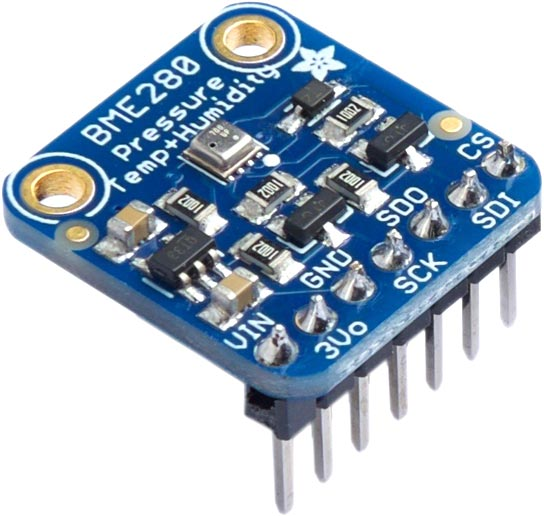## BME280 Humidity Sensor

The Bosch BME280  adds humidity measurement to the BMP280 Pressure Sensor. It measures humidity with ±3 % accuracy, barometric pressure with ±1 hPa absolute accuracy, and temperature with ±1.0 °C accuracy. You can also use it as an altimeter with  ±1 metre or better accuracy. It supports both I2C and SPI interfaces.

It's available on a breakout from Adafruit  or SparkFun .The I2C address is #x77 or 119.

The sensor has a complicated and inconsistent software interface, making the routines longer than for most other comparable sensors.

This sensor requires floating-point calculations, so it's only suitable for 32-bit versions of uLisp. The routines implement the calculations given in the datasheet, but using uLisp's single-precision floating point.

#### Routines

First the following useful routines are defined for reading unsigned and signed integers:

```(defun uint16 (str) (logior (read-byte str) (ash (read-byte str) 8)))

(defun int16 (str) (logior (read-byte str) (ash (ash (read-byte str) 24) -16)))

(defun uint20 (str)
(logior
(logand (ash (read-byte str) -4) #x0F)))```

#### Initialising the sensor

The routine bme280-setup resets the sensor, and then reads the temperature, pressure, and humidity configuration values from the PROM into the lists tcomp, pcomp, and hcomp. You should call this once before using the sensor:

```(defvar tcomp nil)

(defvar pcomp nil)

(defvar hcomp nil)

(defun bme280-setup ()
(with-i2c (str 119)
; Set no oversampling, normal mode
(write-byte #xF4 str)
(write-byte #b00100111 str)
(restart-i2c str)
(write-byte #x88 str)
(restart-i2c str 24)
(setq tcomp (list (uint16 str) (int16 str) (int16 str)))
(setq
pcomp
(list
(uint16 str) (int16 str) (int16 str) (int16 str) (int16 str)
(int16 str) (int16 str) (int16 str) (int16 str)))
(restart-i2c str)
(write-byte #xA1 str)
(restart-i2c str 1)
(restart-i2c str)
(write-byte #xE1 str)
(restart-i2c str 7)
(let ((h2 (int16 str))
(setq
hcomp
(list h1 h2 h3
(logior (ash e4 4) (logand e5 #x0F))
(logior (ash e6 4) (logand (ash e5 -4) #x0F))

The following routine bme280-t returns the temperature in °C. This must be called to set the variable temp-fine before reading the pressure :

```(defvar temp-fine 0)

(defun bmp280-t ()
(let ((tn (lambda (n) (nth (1- n) tcomp))))
(delay 2)
(with-i2c (str 119)
(write-byte #xfa str)
(restart-i2c str 3)
(let* ((temp (uint20 str))
(v1 (* (- (/ temp 16384) (/ (tn 1) 1024)) (tn 2)))
(v1a (- (/ temp 131072) (/ (tn 1) 8192)))
(v2 (* v1a v1a (tn 3)))
(tf (+ v1 v2)))
(setq temp-fine tf)
(/ tf 5120)))))```

For example:

```> (bmp280-t)
22.7207```

It's 22.7°C.

The following routine bme280-p returns the pressure in hPa:

```(defun bme280-p ()
(let ((pn (lambda (n) (nth (1- n) pcomp))))
(with-i2c (str 119)
(write-byte #xf7 str)
(restart-i2c str 3)
(let* ((p (uint20 str))
(v1 (- (/ temp-fine 2) 64000))
(v2 (/ (* v1 v1 (pn 6)) 32768)))
(setq v2 (+ v2 (* v1 (pn 5) 2)))
(setq v2 (+ (/ v2 4) (* (pn 4) 65536)))
(setq v1 (/ (+ (/ (* (pn 3) v1 v1) 524288) (* (pn 2) v1)) 524288))
(setq v1 (* (+ 1 (/ v1 32768)) (pn 1)))
(when (zerop v1) (print "error"))
(setq p (- 1048576 p))
(setq p (/ (* (- p (/ v2 4096)) 6250) v1))
(setq v1 (/ (* (pn 9) p p) 2147483648))
(setq v2 (/ (* p (pn 8)) 32768))
(/ (+ p (/ (+ v1 v2 (pn 7)) 16)) 100)))))```

The following routine bme280-h returns the percentage humidity:

```(defun bme280-h ()
(let ((hn (lambda (n) (nth (1- n) hcomp))))
(with-i2c (str 119)
(write-byte #xfd str)
(restart-i2c str 2)